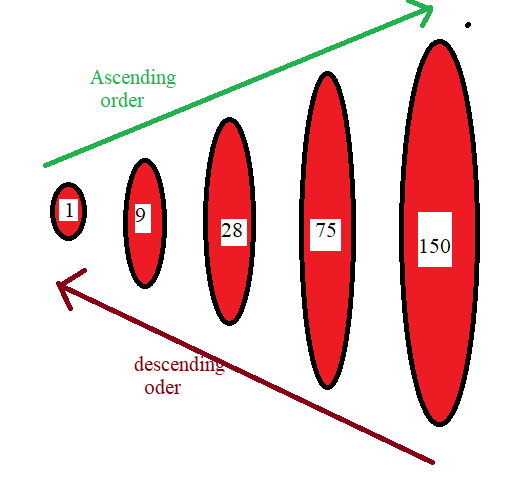QUESTION

# Arrange the following numbers in Descending order -1971, 45321, 88715, 92547.Make ten such examples of Ascending/Descending order and solve them.

Hint: Proceed the solution of this question by starting from the lowest number to the highest number for ascending arrangement while for descending arrangement start from highest number to lowest number.Complete step-by-step solution -
In the question there are given some numbers so to arrange them in ascending order first we select lowest number then 2nd lowest and so on hence accordingly we use sign of greater than and at last we reach to the highest number among them.
similarly to arrange them in descending order first we select highest number then 2nd highest and so on…. hence accordingly we use signs of lesser then and at last we reach to the lowest number among them.

Example-1 Ascending order -1971 < 45321 < 88715 < 92547
Descending order -92547 > 88715 > 45321 > 1971

Example-2 Ascending order -1472 < 55381 < 88715 < 92856
Descending order -92856 > 88715 > 55381 > 1472

Example-3 Ascending order -1878 < 45321 < 78716 < 82548
Descending order -82548> 78716 > 45321 > 1878

Example-4 Ascending order -171 < 321 < 715 < 947
Descending order -947 > 715 > 321 > 171

Example-5 Ascending order -971 < 5321 < 8715 < 9547
Descending order -9547 > 8715 > 5321 > 971

Example-6 Ascending order -1441 < 45521 < 89715 < 99547
Descending order -99547 > 89715 > 45521 > 1441

Example-7 Ascending order -1001 < 40001 < 80005 < 90007
Descending order -90007 > 80005 > 40001 > 1001

Example-8 Ascending order -1771 < 45551 < 88885 < 92227
Descending order -92227 > 88885 > 45551 > 1771

Example-9 Ascending order -91 < 321 < 715 < 927
Descending order -927 > 715 > 321 > 91

Example-10 Ascending order -1989 < 45231 < 88715 < 92599
Descending order -92599 > 88715 > 45321 > 1989

Note: In Mathematics ascending order is a sorting method in which the sort starts from the lowest number to the highest number or the smallest value to the largest value. It means the smallest or earliest value in the order that will come at the first in the list. This order in math helps the children at primary school to learn about the arrangement of numbers or some values in increasing order to solve the problem.
While as we know descending order means to go from biggest to smallest. In alphabets the descending order will be from Z to A or in mathematics higher to lower one.